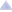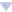Please subscribe to the official Codeforces channel in Telegram via the link https://t.me/codeforces_official. ×

### Enchom's blog

By Enchom, history, 8 years ago,Hello everybody,

It often happens that I have to multiply polynomials quickly and of course FFT is the fastest way to do so. However, I've heard that FFT can have precision errors. I'm not very familiar with the mathematical theory behind it, so I was wondering how can I know whether precision errors will occur and what to do to avoid them. I've also heard about NTT (Number Theoretic Transform) which is similar to FFT but it works with integers only (using modular arithmetics?) but I don't know much about it.

So my questions are :

1) How can I know whether regular FFT using complex numbers would give precise errors, what does it depend on?

2) Is there somewhere I can read about NTT and how it works. I don't have strong mathematical background so something more competitive-programming oriented would be perfect. Additionally, is NTT always precise?

3) Could someone provide quick and simple implementations of FFT/Karatsuba/NTT of simple polynomial multiplication? I've written FFT and Karatsuba before, but my implementations were so bad that they were often outperformed by well-written quadratic solutions.

I do know that these questions were probably asked before so I apologize beforehand. I tried to google but most things I found were math-oriented.Comments (24)
 » wiki may help you:[DFT](https://en.wikipedia.org/wiki/Discrete_Fourier_transform_(general)#Number-theoretic_transform) In this wiki, you can see DFT, FFT, NTT. I think it will give you some benefit.
 » 8 years ago, # | ← Rev. 2 →   I thought this was a nice explanation of NTT: http://www.apfloat.org/ntt.htmlRegarding multiplication with FFT, you can take a look at this, which I wrote for problems MUL and VFMUL.
 » How can I know whether regular FFT using complex numbers would give precise errors, what does it depend on? As a followup, has anyone ever actually had precision problems with their FFT implementation? I've solved quite a bit of problems with mine but I've never had any precision problems. I'm wondering if there are actually people that encountered this problem, or whether this is just a 'fact' that has seeped in from the academic world, where it is more relevant.
•  » » Yes. Double has limited precision, so does long double; if you try to make a convolution of 2 arrays with size 105 and numbers up to 109, you'll get numbers with something like 18 correct digits and 5 random ones at the end.
 » how can I know whether precision errors will occur That's quite simple. Just think about what (complex) FFT computes, what you do to get integers out of it and precision of (long )double. Rule of thumb: modulo size is what matters, not array size. what to do to avoid them Not use FFT, lol. Karatsuba or NTT with multiple modulos (in case the one you want isn't good enough) plus Chinese Remainder Theorem. Additionally, is NTT always precise? It works with integers, sure it is. I've written FFT and Karatsuba before, but my implementations were so bad that they were often outperformed by well-written quadratic solutions. Possible optimisations of Karatsuba: pass references + subarray bounds (convolution(A,B) does convolution(subarray of A,subarray of B) twice, for which you don't need any copying); avoid moduling and subtract instead; therefore, use ints; if you have small arrays, try naive multiplication; that can't be done in ints, but you can keep all numbers of the convolution array somrthing like  < 5mod2 and only subtract 5mod2 when necessary. Loop unrolling might help as well.Some implementations of FFT/NTT should be at e-maxx, and they're fast.Of course, NTT works with primes such that 2x divides p - 1 and 2x is more than your array size. Even if you're not given such a modulo (or aren't given any modulo at all), such primes are quite common — if the resulting values of the convolution fit into int, you can choose such a prime and do NTT. Advantage: no precision errors.
•  » » So say I have to find the convolution of arrays with size 105 and I have to do that modulo 109 + 7. Seems like NTT is the best way to go. The most straight-forward way seems to be to compute it with 3 (?) different primes and then use Chinese Remainder Theorem. However as the values themselves may overflow long long I'd have to use big integers for CRT. Is there any more elegant way to get everything modulo 109 + 7 without coding big numbers?
•  » » » Bigints shouldn't be a problem if you can do NTT... and it's not like it costs a lot of runtime.
•  » » » » Okay, thanks a lot for the help. Finally I wanted to ask is the speed of NTT significantly slower than FFT because in the end of this article they mention it is slower, but I have no idea how much slower.
•  » » » However as the values themselves may overflow long long I'd have to use big integers for CRT. Is there any more elegant way to get everything modulo 109  +  7 without coding big numbers? Sure, when restoring the actual values by CRT you just do all the calculations modulo 109 + 7 and that's it. What's the difference between [do some additions and multiplications (and we only need those to restore the values by CRT) and then take the result modulo 109 + 7] and [do everything modulo 109 + 7 from the very beginning]? Right, there is no difference :)
•  » » » » 8 years ago, # ^ | ← Rev. 2 →   Well I was looking at it here and it seemed like you have to perform division too.Edit: Nevermind, I guess we could also divide modulo the prime.. :D
•  » » » » » This might be helpful. You don't need big integers there until the very end, where you perform only additions and multiplications.
•  » » » » » » Actually, there is a nicer solution without CRT and BigInteger. Think about spliting each number in upper and lower half. Then use something very similar to karatsuba. By only splitting you might get it with 4 convolutions so still O(NlogN) but you can get it down to 3 applying the same concept as Karatsuba. I solved a problem in NlogNlogN using it. And it that 4 to 3 optimization did have noticeable effect. About splitting it is that you choose something like Sqrt(MOD) and split both arrays in higher bits and lower bits. Then use FFT without losing presicion since numbers are of size at most sqrt(MOD). The merge them and there you go.
•  » » » » » » » This algorithm looks to be exactly what Lien posted below.The CRT method doesn't need BigIntegers, and when I tried to program CRT it was slightly faster than the code linked to below. Maybe your Karatsuba implementation is faster than that one, FFTs vary a lot...
•  » » » » » » » » Oh, lol very true. I just figured it out in the Hackerrank that is running. Could you provide a code or submission for the CRT approach i've never used that. I still don't understand very well the idea. And yes now i tested it, and that implementations i kind of slower than mine, athough not for much.
•  » » » » » » » » » There's not much to it, really; you just do the multiplication with 3 different mods and join them afterwards using CRT. Here's a submission that uses it:https://www.hackerrank.com/rest/contests/w23/challenges/sasha-and-swaps-ii/hackers/ffao/download_solution
•  » » » » » » » » » "Access Denied"
•  » » » » » » » » » You need to solve it first to see it.
•  » » » » Sorry for necroposting, but have you ever managed to make this work or was this just an idea?CRT for $x \equiv r_1 \pmod{m_1}, \\ \dots \\ x \equiv r_n \pmod{m_n}$effectively computes $x \equiv r_1 \alpha_1 + \dots + r_n \alpha_n \pmod{m_1 \dots m_n}$for various precomputed $\alpha_i$, but the naively computed sum $r_1 \alpha_1 + \dots + r_n \alpha_n$ might exceed $m_1 \dots m_n$, which we won't be able to notice and fix if we perform multiplication and addition modulo a smaller number than $m_1 \dots m_n$ (e.g. $10^9 + 7$) in this case, no?
•  » » » » » I think you should have no problems like this if you use Garner's algorithm.
•  » » » » » » 7 days ago, # ^ |0.ComplaintFrame { display: inline-block; position: absolute; top: 0; right: -1.4em; } .ComplaintFrame a { text-decoration: none; color: #ff8c00; opacity: 0.5; } .ComplaintFrame a:hover { opacity: 1; } ._ComplaintFrame_popup p, ._ComplaintFrame_popup button { margin-top: 1rem; } ._ComplaintFrame_popup input[type=submit] { padding: 0.25rem 2rem; } ._ComplaintFrame_popup ul { margin-top: 1em !important; margin-bottom: 1em !important; } Yeah, indeed that seems to work. Thanks!
 » There is an easy technique I always use when writing FFT to check precision. res[i] = (int)(a[i].real() + 0.5) error = max(error, abs(a[i].real() - (int)(a[i].real() + 0.5))) This way you can tune your constants, and when error < 1e-3` you can be sure that all rounding is correct.BTW it can be also used in some geometry problems, although not always that trivially.
 » Section 10.4 (page 75) and especially section 10.4.2 (page 76) in this document Johan Håstad's notes for the course Advanced Algorithms might shed some light on what you want to know, though the explanations are quite mathematical...
 » 8 years ago, # | ← Rev. 3 →   Enchom I found on Petr's blog a more convenient way to take FFT mod an arbitrary number. View the comments in Russian mode. For short, the code.